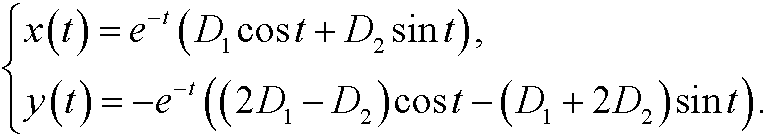# 8. The general solution of a linear system.

Consider the linear dynamical systemthat isWe look for solutions on the formIf we instert these to the system we getthat isThis equation system has nontrivial solutions if and only ifthat is, if and only ifThis is the characteristic equation of the system and the solutionsits eigenvalues. If we now for each eigenvaluessolve the equation system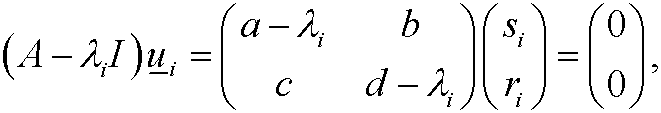we obtain the eigenvectorsThen we have the general solution of our original dynamical system as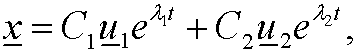that iswhere C1 and C2 are arbitrary constants.

Example 8:
Assume that the matrix A isThis matrix has characteristic equationwith the eigenvalues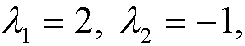and corresponding eigenvectors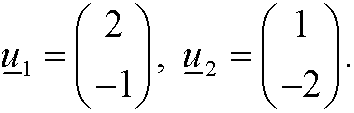This means that the general solution of the corresponding dynamical system isthat isExample 9: Solve the systemSolution: We consider the matrix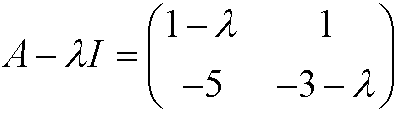and its characteristic equationthat isThe eigenvalues thus arewith corresponding eigenvectorsThis means that the dynamical system has the general solutionthat isThese are all complex solutions. We are actually only interested in the real solutions. With help of Euler's formula we get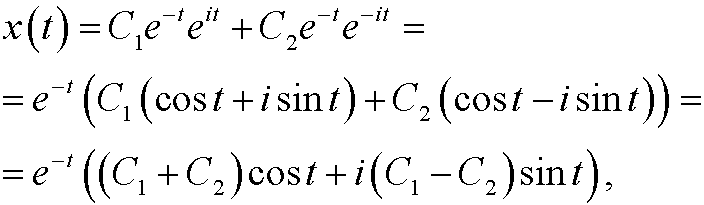If we now pick arbitrary real constants D1 and D2 and putwe get the general real solution